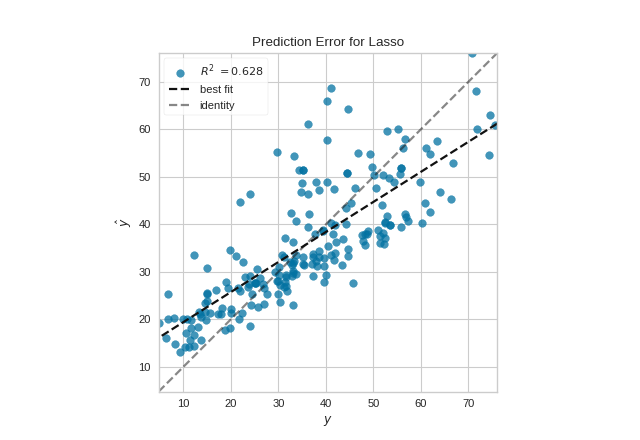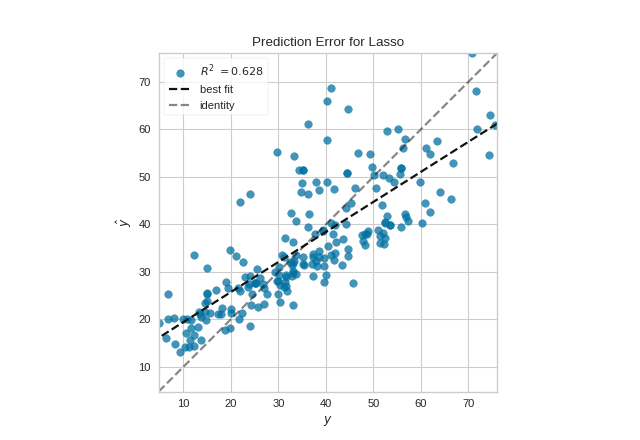# Prediction Error Plot¶

A prediction error plot shows the actual targets from the dataset against the predicted values generated by our model. This allows us to see how much variance is in the model. Data scientists can diagnose regression models using this plot by comparing against the 45 degree line, where the prediction exactly matches the model.

 Visualizer PredictionError Quick Method prediction_error() Models Regression Workflow Model Evaluation
from sklearn.linear_model import Lasso
from sklearn.model_selection import train_test_split

from yellowbrick.regressor import PredictionError

# Create the train and test data
X_train, X_test, y_train, y_test = train_test_split(X, y, test_size=0.2, random_state=42)

# Instantiate the linear model and visualizer
model = Lasso()
visualizer = PredictionError(model)

visualizer.fit(X_train, y_train)  # Fit the training data to the visualizer
visualizer.score(X_test, y_test)  # Evaluate the model on the test data
visualizer.show()                 # Finalize and render the figure## Quick Method¶

The same functionality can be achieved with the associated quick method prediction_error. This method will build the PredictionError object with the associated arguments, fit it, then (optionally) immediately show the visualization.

from sklearn.linear_model import Lasso
from sklearn.model_selection import train_test_split

from yellowbrick.regressor import prediction_error

# Create the train and test data
X_train, X_test, y_train, y_test = train_test_split(X, y, test_size=0.2, random_state=42)

# Instantiate the linear model and visualizer
model = Lasso()
visualizer = prediction_error(model, X_train, y_train, X_test, y_test)## API Reference¶

Comparison of the predicted vs. actual values for regression problems

class yellowbrick.regressor.prediction_error.PredictionError(model, ax=None, shared_limits=True, bestfit=True, identity=True, alpha=0.75, is_fitted='auto', **kwargs)[source]

Bases: yellowbrick.regressor.base.RegressionScoreVisualizer

The prediction error visualizer plots the actual targets from the dataset against the predicted values generated by our model(s). This visualizer is used to detect noise or heteroscedasticity along a range of the target domain.

Parameters
modela Scikit-Learn regressor

Should be an instance of a regressor, otherwise will raise a YellowbrickTypeError exception on instantiation. If the estimator is not fitted, it is fit when the visualizer is fitted, unless otherwise specified by is_fitted.

axmatplotlib Axes, default: None

The axes to plot the figure on. If None is passed in the current axes will be used (or generated if required).

shared_limitsbool, default: True

If shared_limits is True, the range of the X and Y axis limits will be identical, creating a square graphic with a true 45 degree line. In this form, it is easier to diagnose under- or over- prediction, though the figure will become more sparse. To localize points, set shared_limits to False, but note that this will distort the figure and should be accounted for during analysis.

bestfitbool, default: True

Draw a linear best fit line to estimate the correlation between the predicted and measured value of the target variable. The color of the bestfit line is determined by the line_color argument.

identitybool, default: True

Draw the 45 degree identity line, y=x in order to better show the relationship or pattern of the residuals. E.g. to estimate if the model is over- or under- estimating the given values. The color of the identity line is a muted version of the line_color argument.

alphafloat, default: 0.75

Specify a transparency where 1 is completely opaque and 0 is completely transparent. This property makes densely clustered points more visible.

is_fittedbool or str, default=’auto’

Specify if the wrapped estimator is already fitted. If False, the estimator will be fit when the visualizer is fit, otherwise, the estimator will not be modified. If ‘auto’ (default), a helper method will check if the estimator is fitted before fitting it again.

kwargsdict

Keyword arguments that are passed to the base class and may influence the visualization as defined in other Visualizers.

Notes

PredictionError is a ScoreVisualizer, meaning that it wraps a model and its primary entry point is the score() method.

Examples

>>> from yellowbrick.regressor import PredictionError
>>> from sklearn.linear_model import Lasso
>>> model = PredictionError(Lasso())
>>> model.fit(X_train, y_train)
>>> model.score(X_test, y_test)
>>> model.show()

Attributes
score_float

The R^2 score that specifies the goodness of fit of the underlying regression model to the test data.

draw(y, y_pred)[source]
Parameters
yndarray or Series of length n

An array or series of target or class values

y_predndarray or Series of length n

An array or series of predicted target values

Returns
axmatplotlib Axes

The axis with the plotted figure

finalize(**kwargs)[source]

Finalizes the figure by ensuring the aspect ratio is correct and adding the identity line for comparison. Also adds a title, axis labels, and the legend.

Parameters
kwargs: generic keyword arguments.

Notes

Generally this method is called from show and not directly by the user.

score(X, y, **kwargs)[source]

The score function is the hook for visual interaction. Pass in test data and the visualizer will create predictions on the data and evaluate them with respect to the test values. The evaluation will then be passed to draw() and the result of the estimator score will be returned.

Parameters
Xarray-like

X (also X_test) are the dependent variables of test set to predict

yarray-like

y (also y_test) is the independent actual variables to score against

Returns
scorefloat
yellowbrick.regressor.prediction_error.prediction_error(model, X_train, y_train, X_test=None, y_test=None, ax=None, shared_limits=True, bestfit=True, identity=True, alpha=0.75, is_fitted='auto', show=True, **kwargs)[source]

Quickly plot a prediction error visualizer

Plot the actual targets from the dataset against the predicted values generated by our model(s).

This helper function is a quick wrapper to utilize the PredictionError ScoreVisualizer for one-off analysis.

Parameters
modelthe Scikit-Learn estimator (should be a regressor)

Should be an instance of a regressor, otherwise will raise a YellowbrickTypeError exception on instantiation. If the estimator is not fitted, it is fit when the visualizer is fitted, unless otherwise specified by is_fitted.

X_trainndarray or DataFrame of shape n x m

A feature array of n instances with m features the model is trained on. Used to fit the visualizer and also to score the visualizer if test splits are not directly specified.

y_trainndarray or Series of length n

An array or series of target or class values. Used to fit the visualizer and also to score the visualizer if test splits are not specified.

X_testndarray or DataFrame of shape n x m, default: None

An optional feature array of n instances with m features that the model is scored on if specified, using X_train as the training data.

y_testndarray or Series of length n, default: None

An optional array or series of target or class values that serve as actual labels for X_test for scoring purposes.

axmatplotlib Axes

The axes to plot the figure on.

shared_limitsbool, default: True

If shared_limits is True, the range of the X and Y axis limits will be identical, creating a square graphic with a true 45 degree line. In this form, it is easier to diagnose under- or over- prediction, though the figure will become more sparse. To localize points, set shared_limits to False, but note that this will distort the figure and should be accounted for during analysis.

bestfitbool, default: True

Draw a linear best fit line to estimate the correlation between the predicted and measured value of the target variable. The color of the bestfit line is determined by the line_color argument.

identity: bool, default: True

Draw the 45 degree identity line, y=x in order to better show the relationship or pattern of the residuals. E.g. to estimate if the model is over- or under- estimating the given values. The color of the identity line is a muted version of the line_color argument.

alphafloat, default: 0.75

Specify a transparency where 1 is completely opaque and 0 is completely transparent. This property makes densely clustered points more visible.

is_fittedbool or str, default=’auto’

Specify if the wrapped estimator is already fitted. If False, the estimator will be fit when the visualizer is fit, otherwise, the estimator will not be modified. If ‘auto’ (default), a helper method will check if the estimator is fitted before fitting it again.

show: bool, default: True

If True, calls show(), which in turn calls plt.show() however you cannot call plt.savefig from this signature, nor clear_figure. If False, simply calls finalize()

kwargsdict

Keyword arguments that are passed to the base class and may influence the visualization as defined in other Visualizers.

Returns
axmatplotlib Axes

Returns the axes that the prediction error plot was drawn on.